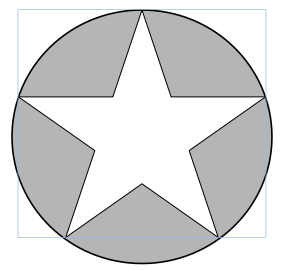High School Math : Geometry

Example Questions

If a circle has a circumference of 16π, what would its area be if its radius were halved?

64π

16π

16π

Explanation:

The circumference of a circle = πd where d = diameter.  Therefore, this circle’s diameter must equal 16.  Knowing that diameter = 2 times the radius, we can determine that the radius of this circle = 8.  Halving the radius would give us a new radius of 4.  To find the area of this new circle, use the formula A=πr² where r = radius.  Plug in 4 for r.  Area will equal 16π.A star is inscribed in a circle with a diameter of 30, given the area of the star is 345, find the area of the shaded region, rounded to one decimal.

361.5

356.5

341.5

346.5

351.5

361.5

Explanation:

The area of the circle is (30/2)2*3.14 (π) = 706.5, since the shaded region is simply the area difference between the circle and the star, it’s 706.5-345 = 361.5

Example Question #11 : Plane Geometry

The diameter of a circle increases by 100 percent. If the original area is 16π, what is the new area of the circle?

50π

54π

160π

64π

49π

64π

Explanation:

The original radius would be 4, making the new radius 8 and by the area of a circle (A=π(r)2) the new area would be 64π.

Example Question #1 : How To Find The Area Of A Circle

A circle with a diameter of 6” sits inside a circle with a radius of 8”.  What is the area of the interstitial space between the two circles?

25π in2

55π in2

7π in2

28π in2

72π in2

55π in2

Explanation:

The area of a circle is πr2

The diameter of the first circle = 6” so radius of the first circle = 3” so the area = π * 32 = 9π in2

The radius of the second circle = 8” so the area = π * 82 = 64π in2

The area of the interstitial space = area of the first circle – area of the second circle.

Area = 64π in2  - 9π in2 = 55π in2

If the radius of a circle is tripled, and the new area is 144π what was the diameter of the original circle?

12

6

4

8

7

8

Explanation:

The area of a circle is A=πr2. Since the radius was tripled 144π =π(3r)2. Divide by π and then take the square root of both sides of the equal sign to get 12=3r, and then r=4. The diameter (d) is equal to twice the radius so d= 2(4) = 8.

Example Question #541 : Act Math

If the radius of Circle A is three times the radius of Circle B, what is the ratio of the area of Circle A to the area of Circle B?

15

12

3

6

9

9

Explanation:

We know that the equation for the area of a circle is π r2. To solve this problem, we pick radii for Circles A and B, making sure that Circle A’s radius is three times Circle B’s radius, as the problem specifies. Then we will divide the resulting areas of the two circles. For example, if we say that Circle A has radius 6 and Circle B has radius 2, then the ratio of the area of Circle A to B is: (π 62)/(π 22) = 36π/4π. From here, the π's cancel out, leaving 36/4 = 9.

Example Question #541 : Act Math

1. A circle is inscribed inside a 10 by 10 square. What is the area of the circle?

40π

50π

25π

10π

100π

25π

Explanation:

Area of a circle = A = πr2

R = 1/2d = ½(10) = 5

A = 52π = 25π

Example Question #11 : How To Find The Area Of A Circle

A square has an area of 1089 in2. If a circle is inscribed within the square, what is its area?

272.25π in2

33π in2

16.5 in2

1089π in2

33 in2

272.25π in2

Explanation:

The diameter of the circle is the length of a side of the square. Therefore, first solve for the length of the square's sides. The area of the square is:

A = s2 or 1089 = s2. Taking the square root of both sides, we get: s = 33.

Now, based on this, we know that 2r = 33 or r = 16.5. The area of the circle is πr2 or π16.52 = 272.25π.

Example Question #11 : How To Find The Area Of A Circle

A square has an area of 32 in2. If a circle is inscribed within the square, what is its area?

8π in2

32π in2

16π in2

4√2 in2

2√2 in2

8π in2

Explanation:

The diameter of the circle is the length of a side of the square. Therefore, first solve for the length of the square's sides. The area of the square is:

A = s2 or 32 = s2. Taking the square root of both sides, we get: s = √32 = √(25) = 4√2.

Now, based on this, we know that 2r = 4√2 or r = 2√2. The area of the circle is πr2 or π(2√2)2 = 4 * 2π = 8π.

Example Question #61 : Circles

A manufacturer makes wooden circles out of square blocks of wood. If the wood costs \$0.25 per square inch, what is the minimum waste cost possible for cutting a circle with a radius of 44 in.?

5808 dollars

1936 dollars

1936π dollars

7744 – 1936π dollars

1936 – 484π dollars